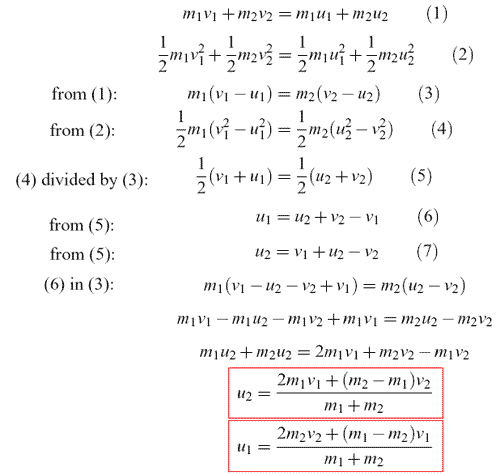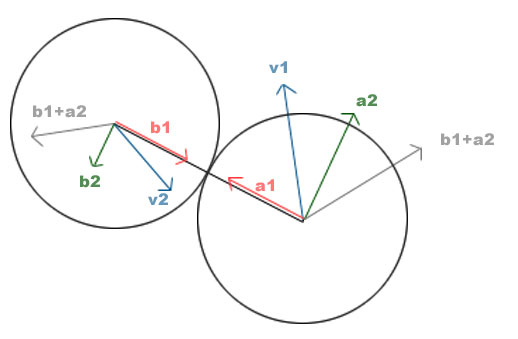Simple Collision of Balls (1- / 2- dimensional) - by Sunshine

Part I - Introduction

Some time ago I started to code a little 2D Billard game. Well unfortunately I must admit I have stopped it, but I have dealed a little bit with two-dimensional collision of balls, so I thought why not sharing what I've learned. Cause it was not too easy to find really good information about this topic...
First we start with one-dimensional collision, cause we can reduce the two-dim case to the one-dim one. I want to emphasize that it's whether the best nor the 100% correct approach but it's works and it's not too bad.
We proceed from the following assumptions:
- the collsion is fully elastic (no energy will be lost)
- the balls have no inner rotation
- the body of a ball is homogenous (means the mass is spread evenly over the whole body).

Some notes: we talk about vectors and not about numbers, although I write them without these little arrows. Second, I don't know if the 2D approach I figured out is correct, but it looks not that bad... so don't flame me if I did some mistakes :-(

Part II - 1D Collision

Ok, let's start :-) First thing we have to know is Newton's principle of conservation of momentum: It states that when a system of rigid bodies collide, momentum is conserved. Here it means the sum of the pulses of the balls colliding remains the same.
This also applies for the kinetic energy of the two balls: Conservation of kinetic energy between two colliding bodies means that the sum of kinetic energy of both bodies before impact is equal to the sum of the kinetic energy of both bodies after impact.

Now go to formula: Assume we have two balls: ball1 with mass m1 and ball2 with mass m2. Before collision, ball1 has speed v1 and ball2 v2; after collision the new speed of ball1 is u1 and of ball2 is u2. As stated we have 2 formula:

 Conservation of momentum : m1 * v1 + m2 * v2 = m1 * u1 + m2 * u2

 Conservation of kinetic energy : m1 * (v1)^2 + m2 * (v2)^2 = m1 * (u1)^2 + m2 * (u2)^2

Good! Two formula and 2 unknown we want to know (u1 and u2). So let's solve these equations and we get:Fine! So after we have detected a collision (check if distance of two balls <= radius of ball1 + radius of ball 2) we can easily calculate the new speed values u1 and u2.

Ok, here you see a tiny applet demonstrating what we've learned so far... You can change speed and mass of the balls on the fly by typing values between 0.0 and 10.0 in the textfields and hit enter. Enjoy!
A last note: when you look at the source of this applet, you see something strange in the handlecollision() function: some code to make sure that both balls don't hang in each other (as I have commented it). That's because the real collision normally has occured before you detected that the distance between two center of the balls is less than the sum of those two balls r1+r2. The correct way for solving this issue is to 'rewind' the simulation to find the time when the balls really collide. As you see, I cheated here. On a collision detection, I move the balls step by step apart from each other until they don't hang each other anymore. Well, quite lame, but easy to implement and not that bad as you see...
Ok, knowing how collsion in one dimension works, let's go on to the more interesting two-dimensional case :-)

Part III - 2D Collision

So, let's continue with the more interesting case. The conservation of momentum and kinetic energy are still valid (logical, physics don't change...), but the problem is that we don't know the direction in which the balls will move after a collision. But we didn't talk about 1D collision for nothing... we can reduce the 2D case into a 1D one and with some vector calculating we are finished. So let's consider the following picture:Here you see the situation when ball1 with velocity v1 collide with ball2 with velocity v2. First we calculate the values of the new speed vectors. As said before, we reduce it to the one-dimensional case.
So find the connecting line between both balls and call this vector v. Now we can calculate the component of v1 in the direction of v with a simple dotproduct. Before that we normalize v and v1, because then following rule obtains: DotProduct(v, v1) = cos(angle between both vectors). Multiply the result with the normalied vector of v and you get what you want: a1. Repeat this step with v2 to get b1. So now you have the one-dimensional case like above with the vectors a1 and b1 and you can calculate the new velocities.
Some pseudocode:

 Vector2D v = center(ball1) - center(ball2); v.normalize; Vector2D va = v1.normalize; Vector2D vb = v2.normalize; double va1 = DotProduct(v, va); double va2 = DotProduct(v, vb); va1 = va1 * Ball1.speed;              // va1 = |a1| va2 = va2 * Ball2.speed;              // va2 = |b1| // now calculate with va1 and va2 the new velocities like the 1D case...

So at this point we have the values of the new velocities but not the direction. Here comes some easy vector calculation. We know how we get vectors a1 and b1, so with some vector addition we can calculate a2 and b2 and suddenly we can calc the final (gray) vectors. Again some pseudocode:

 Vector2D a1 = v * Vector2D.dotProduct(v, va); a1 = a1 * speed(ball1); Vector2D a2 = Ball1.speed - a1; Vector2D b1 = v * Vector2D.dotProduct(v, vb); b1 = b1 * speed(ball2); Vector2D b2 = Ball2.speed - b1; a1 = a1 * newspeed(ball1); // apply new speed value to a1 b1 = b2 * newspeed(ball2); // apply new speed value to b1 Vector2D finalBall1vector = b1 + a2; Vector2D finalBall2vector = a1 + b2;

Well, that's fine. Here the applet showing what we've learned. Again you can change speed and mass values and the fly. If you pause the simulation, you can even drag the balls with the mouse to new positions...

Questions, criticism? Mail me!

Sunshine, May 2006

This site is part of Sunshine's Homepage